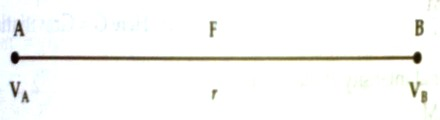# Relation between Intensity and Potential Difference

Relation between intensity and potential difference

In order to establish the relation between gravitational intensity and gravitational potential let A and B be two very close points in the gravitational field and let the distance between them be r [Figure]. Let the potential at A = VA and that at B = VB. Since the two points are very close to each other, so we can consider the constant gravitational field there. Let the intensity of the field = F.Now, amount of work done in bringing a unit mass from point B to point A = Force x distance = Intensity x distance

= F x AB = E x r       [F = E for unit mass]

This is the potential difference between point A and B that is (VA – VB)

so, F x AB = (VA – VB)

or, F = (VA – VB)/AB = (VA – VB)/r

i.e., rate of change of potential difference with distance is called intensity.

If displacement towards the direction of field is AB = dr and potential at point A is VA and that at point B is VB, then VA – VB = – dV

So, F = – dV/dr

This is the relation between gravitational intensity and potential difference.